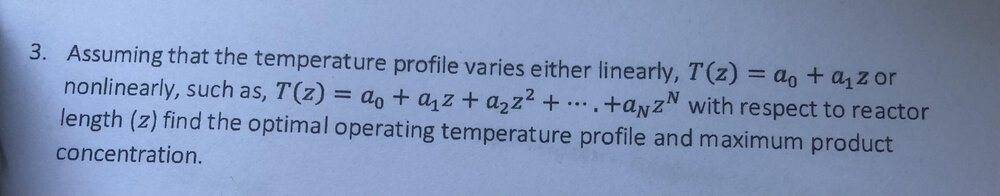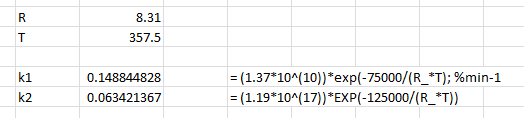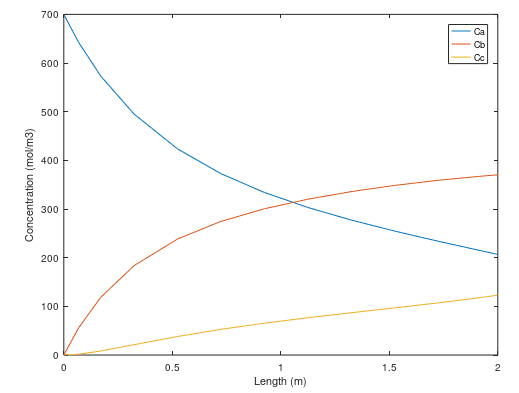# MATLAB Plug flow reactor optimisation Problem

• MATLAB
Will26040
Summary
I am trying to create MATLAB code that will find the optimal operating temperature and maximum product concentration in a PFR. I have included my current attempt.
The assignment is to find the optimal operating temperature and maximum product concentration of reactant B, assuming a constant temperature across the PFR length. Please could someone help? thanks

the reaction is a series reaction: A → B → C (liquid phase)

Here is my current code which is providing T as the initial guess value:

function Main
x0 = 
OPTIONS = optimoptions('fmincon','Display','iter');
x = fminunc(@fun,x0,OPTIONS)
end

function obj = fun(x)
T = x(1);
Lspan = [0 2]; %reactor 2m long
y0 = [700 0 0];
[L,y] = ode15s(@(L,y) PFR1(L,y,T),Lspan,y0);
obj = -y(end,2)
end

function f = PFR1(L,y,T)
%series reactions Y is the concentrations of species and V PFR volume
Ca= y(1);
Cb= y(2);
Cc= y(3);

%Parameters
R = 8.31; %J.mol-1K-1
u = 0.25; %m.min-1

%rate constants
k1 = (1.37*10^(10))*exp(-75000/R*T); %min-1
k2 = (1.19*10^(17))*exp(-125000/R*T); %min-1

%Reaction Rates
ra = -k1*Ca;
rb = k1*Ca-k2*Cb
rc = k2*Cb;;

%Differential Equations
dCbdL = rb/u;
dCcdL = rc/u;

f = [dCadL; dCbdL; dCcdL]

end

## Answers and Replies

Arjan82
Two things:
- could you use a codeblock for Matlab code? That makes everything much easier to read
- what exactly do you want help with? Apparently this doesn't work? what is going wrong?

•Will26040
Will26040
yep sorry didnt know about code blocks:

Matlab:
function Main
x0 = 
OPTIONS = optimoptions('fmincon','Display','iter');
x = fminunc(@fun,x0,OPTIONS)
end

function obj = fun(x)
T = x(1);
Lspan = [0 2]; %reactor 2m long
y0 = [700 0 0];
[L,y] = ode15s(@(L,y) PFR1(L,y,T),Lspan,y0);
obj = -y(end,2)
end

function f = PFR1(L,y,T)
%series reactions Y is the concentrations of species and V PFR volume
Ca= y(1);
Cb= y(2);
Cc= y(3);

%Parameters
R = 8.31; %J.mol-1K-1
u = 0.25; %m.min-1

%rate constants
k1 = (1.37*10^(10))*exp(-75000/R*T); %min-1
k2 = (1.19*10^(17))*exp(-125000/R*T); %min-1

%Reaction Rates
ra = -k1*Ca;
rb = k1*Ca-k2*Cb
rc = k2*Cb;;

%Differential Equations
dCbdL = rb/u;
dCcdL = rc/u;

f = [dCadL; dCbdL; dCcdL]

end

It doesnt seem to be working at the moment as it just gives me whatever I put in for x0 as the optimal temperature. The task is to find the optimal temperature (minimum) that gives the maximum Cb value. Am I going about it right? thanks

Arjan82
Have you tried the Matlab debugger? It helps in figuring if the in-between steps work. Now, I'm not a chemist, but these are the things that I would check:
- exp(-75000/R*T) (and the other exp as well) is always zero, after googling a bit seems like it should be exp(-75000/R/T). This explains the insensitivity on x0.
- Even after this, the value of the two k's are extremely small (9e-30 or so), which seems incorrect, or if not, then it will lead to all sorts of numerical trouble. Is this 75000 (activation energy?) correct?
- in the formula for k, the value of (1.37*10^(10)) seems very large, is this the molar concentration? Which you also specify in y0? there it is in the order of a few 100, not 10 billion.

So, that's where I would start, good luck!

•Will26040 and BvU
Homework Helper
Not a chemist either, but
• I second @Arjan82: ##\ \ e^{-E_a/(RT)}##
• T = 100 K is way too low a temperature to get the reaction going• You have ra = -k1*Ca; so you don't want dCadL = -ra/u; but dCadL = +ra/u;
• What about L ?

Last edited:
•Will26040
Will26040
thanks to you both for the help, I think I've sorted it with your advice.

Homework Helper
I don't have MatLab, so I fumble with Octave (And with excel) found about 357.5 K.
Did you have to make any other changes ?

•Will26040
Will26040
I don't have MatLab, so I fumble with Octave (And with excel) found about 357.5 K.
Did you have to make any other changes ?
thanks thats what I got too. I didnt have to make any other changes

•BvU
Will26040
I now have a second part which I was wondering if anyone could help me please. You have to assume that the temperature profile varies nonlinearly with length (z) of the reactor:
##T(z)=a+bz+cz^2##
I have some code for it however I think it is wrong:

Matlab:
A=[-1 0 0 ]; B=;
Aeq=[]; Beq=[];
LB=[]; UB=[];
Nonlcon=[];
T = 350;
a = -1;
b = -1;
x0 = [T a b];
options = []
x = fmincon(@fun,x0,A,B,Aeq,Beq,LB,UB,Nonlcon,options)

function obj = fun(x)
T = x(1);
a = x(2);
b = x(3);
zspan = [0 2]; % Reactor 2m long
y0 = [700 0 0]; % Initial values for species concentrations [Ca Cb Cc]
[z, y] = ode45(@(z,y) PFR2(z,y,T,a,b),zspan,y0);
obj = -y(end,2)
T1 = x(1)+(x(2).*z)+(x(3).*z.^(2));
plot(z,y(:,1),z,y(:,2),z,y(:,3))
xlabel('Length (m)');
ylabel('Concentration (mol/m3)');
legend('Ca', 'Cb', 'Cc');

end

function f = PFR2(z,y,T,a,b)
Ca = y(1);
Cb = y(2)
Cc = y(3);
T=T+(a*z)+(b*(z^2));

%Parameters
R = 8.31; %J.mol-1K-1
u = 0.25; %m.min-1

%rate constants
k1 = (1.37*10^(10))*exp(-75000/(R*T)); %min-1
k2 = (1.19*10^(17))*exp(-125000/(R*T)); %min-1

%Reaction Rates
ra = k1*Ca;
rb = k1*Ca-k2*Cb;
rc = k2*Cb;

%Differential Equations
%dTdz = a+(b*(z^2))
dCbdz = rb/u;
dCcdz = rc/u;

f = [dCadz; dCbdz; dCcdz]
end

Im not sure if im going about it in the right way. Should the equation for how T varies with length be a nonlinear equality constraint? thanks

Last edited:
Homework Helper
Should the equation for how T varies with length be a nonlinear equality constraint?
Not a matlab (or octave) expert, but T is given and doesn't constrain anything.
But you can't have both Y and z for the same variable. I would replace T=T+(a*z)+(b*(z^2)); by T=T+(a*y)+(b*(y^2));...

##\ ##

Will26040
okay thanks for all the help so far. Do you think it mostly looks right other than that?

Homework Helper
Should be OK, yes -- but that is no guaranteeIs this going towards a gradually more detailed model with e.g. an exothermal reaction ? and then some cooling on the outside ?##\ ##

Will26040
Should be OK, yes -- but that is no guaranteeIs this going towards a gradually more detailed model with e.g. an exothermal reaction ? and then some cooling on the outside ?##\ ##
Thanks. This is the last question that involves coding, however, it does say you can consider the energy balance at the end.

I think something is wrong with my code. I only seem to get one temperature in the output of x, but im meant to be getting a temperature profile so that I can plot a graph of temperature vs length of reactor

Homework Helper
You do the plotting inside the objective function ? That function can be called a zillion times !

##\ ##

Homework Helper
And the temerature profile is given as far as I could see ? ##T(z)=350 - z - z^2##

Homework Helper
Ah, complete misunderstanding at my end. What is it you now want to optimize ? Only ##z## (before it was T) it seems ?

Will26040Hi, thanks for your patience. I have attached the actual question; it still is T that I want to optimize, however you have to assume it varies non-linearly with the length- which is fixed at 2m. I have assumed the relationship
##T(z)=a+bz+cz^2##
Im not sure if I am doing it right.

Homework Helper
- Even after this, the value of the two k's are extremely small (9e-30 or so), which seems incorrect, or if not, then it will lead to all sorts of numerical trouble
Will26040 said:
I was just wondering in the post that you helped me with you said 'T = 100 K is way too low a temperature to get the reaction going' I was just wondering how you knew this? I think I'm meant to derive some constraints or something. thanks
I simply calculated a few k valuesInspired by previous painfulexperience mixing up temperatures in C and K.

In numerical solvers and such it is indeed often a good idea to restrict the range of ##T## and to work with a $$k_0' = k_0\, e^{-{Ea\over RT_0}}$$ that isn't so huge so that $$k(T) = k_0' \, e^{-{Ea\over R}({1\over T}-{1\over T_0})}$$to avoid problems with the argument of exponentiation.

##\ ##

Ah, complete misunderstanding at my end. What is it you now want to optimize ? Only ##z## (before it was T) it seems ?
That wasn't too good a shot either. You optimize ##x = [T, a, b] ## !

So I tried your code from #9. With a few mods it runs in Octave
Matlab:
function main %(pkg load optim can be done in command window)
A=[-1 0 0 ]; B=;
Aeq=[]; Beq=[];
LB=[]; UB=[];
Nonlcon=[];
T = 350;
a = -1;
b = -1;
x0 = [T a b];
options = optimset();
%x = fminunc(@fun,x0,A,B,Aeq,Beq,LB,UB,Nonlcon,options)
x = fminunc(@fun,x0,options)
end

In short: ihave no idea what A,B,Aeq,Beq,LB,UB,Nonlcon, are supposed to do.

But weven without those, it runs and terminates with a pictureand
Code:
f =

-97.503
33.805
63.698

obj = -370.26
x =

369.0975   -21.3115     6.9389

>>
which may or may not be a reasonable outcome.

I would expect you need to bring in other cosiderations to deal with question 3. After all, yield is one single number and optimizing ##a_i \ \ i \in (0....N)\ ## is N dimensional. Don't know how to tackle that.

When I look at the profile: I kind of expect ##C_b## at 2m to be at a maximum is equivalent to ##r_b =0\ ## at that point, but the figure doesn't show that.

I may find the time to plod through a book on reaction engineering at some point, but at the moment I can't help much further I'm afraid.

##\ ##

Will26040
I simply calculated a few k values
Inspired by previous painfulexperience mixing up temperatures in C and K.

In numerical solvers and such it is indeed often a good idea to restrict the range of ##T## and to work with a $$k_0' = k_0\, e^{-{Ea\over RT_0}}$$ that isn't so huge so that $$k(T) = k_0' \, e^{-{Ea\over R}({1\over T}-{1\over T_0})}$$to avoid problems with the argument of exponentiation.

##\ ##

That wasn't too good a shot either. You optimize ##x = [T, a, b] ## !

So I tried your code from #9. With a few mods it runs in Octave
Matlab:
function main %(pkg load optim can be done in command window)
A=[-1 0 0 ]; B=;
Aeq=[]; Beq=[];
LB=[]; UB=[];
Nonlcon=[];
T = 350;
a = -1;
b = -1;
x0 = [T a b];
options = optimset();
%x = fminunc(@fun,x0,A,B,Aeq,Beq,LB,UB,Nonlcon,options)
x = fminunc(@fun,x0,options)
end

In short: ihave no idea what A,B,Aeq,Beq,LB,UB,Nonlcon, are supposed to do.

But weven without those, it runs and terminates with a picture
and
Code:
f =

-97.503
33.805
63.698

obj = -370.26
x =

369.0975   -21.3115     6.9389

>>
which may or may not be a reasonable outcome.

I would expect you need to bring in other cosiderations to deal with question 3. After all, yield is one single number and optimizing ##a_i \ \ i \in (0....N)\ ## is N dimensional. Don't know how to tackle that.

When I look at the profile: I kind of expect ##C_b## at 2m to be at a maximum is equivalent to ##r_b =0\ ## at that point, but the figure doesn't show that.

I may find the time to plod through a book on reaction engineering at some point, but at the moment I can't help much further I'm afraid.

##\ ##
Thanks for all your help! that's all very useful info. Ill get back to you if I find a better solution